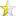Mar 9, 2012 (last update: Mar 22, 2012)## Pointers Simplified:

As the name says a pointer is a special type of variable which is used to point at another variable/pointer.

### Declare a pointer:

Pointer variables are declared by prefixing with * symbol.
 ``1234`` `````` // pointervariablename; int *gunpointer=0; int* gunpointer=0; float* fp;``````

now lets declare some variables to point at
int ivalue=10;
float fvalue=5.0;

### Pointing the gun/pointer:

 ``12345`` `````` gunpointer=ivalue; /*invalid u cant point the gun without knowing where the person is*/ gunpointer=&ivalue;/*valid now u know the address, u can point at the person residing at that address*/ gunpointer=&fvalue;/*invalid wrong gun choice,ur using a toy gun to rob a bank a pointer can only point to a variable of same type*/``````

### Firing the gun or dereferencing the pointer: (fetching the value from a pointer)

Now once the pointer is pointing to a variable, how do you get the value of pointed location or dereference a pointer?
Simple by using the * mark again
 ``12`` ``````int ivalue1=*gunpointer;//fetches the value stored at that location printf("%d",ivalue1);``````

### Note: * is used in two places

 ``12`` `````` int* ip ;// here it means u are declaring a pointer to an integer. int k=*ip;//or printf(“%d”,*ip); here it means dereferencing or fetching the ``````

value stored at the address pointed by pointer.

### Two dimensional Pointers:

they can be considered as pointers to pointers

#### ex1:pointer to a pointer

 ``123`` `````` char* str="hi im learning pointers"; char** strp=&str; printf("%s",*strp);``````

here strp acts as a pointer to str which is pointing to the starting address of string "hi im learning pointers"

This concept is very useful when an array has to be populated using pass by reference

#### ex2 (complicated ):

 ``1234567891011121314151617181920212223242526272829303132`` ``````#include #include void populatearray(int** mainarray,int* count) { int a[]={1,2,3,4,5}; //create a single dimension array for storing the values int* myarray=new int; //assign the values to be stored to the array for(int i=0;i<5;i++) { myarray[i]=a[i]; } //store the count in the adress pointed by formal parameter *count=5; //store the array in the adress pointed by formal parameter *mainarray=myarray; } void main() { //the main array where values have to be stored int* arraymain=0; //count of elements int maincount=0; //pass the adess of pointer to array, adress of counter populatearray(&arraymain,&maincount); //check whether pass by reference has worked printf("The array Elements:\n"); for(int i=0;i